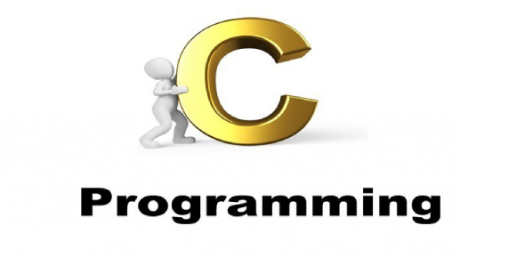# C Programming Language: Basic Trivia Test

5 Questions | Attempts: 85SettingsThis basic trivia test is on C programming language. It is perfect to test out just how much you know about the language. As a future programmer just how good do you think you will score in this quiz, do you have what it takes for the top score? Take up the quiz below and get the perfect answer to it. All the best!

• 1.
What is the output? void main() {         int i;       for(i=0; i  ; i++)       {   i++;  }       printf("%d",i); }
• A.

0

• B.

1

• C.

2

• 2.
What is the output? void main() {       struct tg      {            }; printf("%d",sizeof(tg)); }
• A.

0 bytes

• B.

1 byte

• C.

2 bytes

• 3.
Which of the following operator is not present in c language?
• A.

++

• B.

&&

• C.

***

• 4.
#include <stdio.h> int main() {     int i = 1, 2, 3;          printf("%d", i);          return 0; }
• A.

1

• B.

3

• C.

Garbage value

• D.

Compile time error

• 5.
What is the output of below program? #include <stdio.h> int foo(int* a, int* b) {     int sum = *a + *b;     *b = *a;     return *a = sum - *b; } int main() {     int i = 0, j = 1, k = 2, l;     l = i++ || foo(&j, &k);     printf("%d %d %d %d", i, j, k, l);     return 0; }
• A.

1 2 1 1

• B.

1 1 2 1

• C.

1 2 2 1

• D.

1 2 2 2

## Related TopicsBack to top
×

Wait!
Here's an interesting quiz for you.